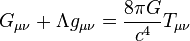# Frame-dragging facts for kids

Kids Encyclopedia Facts
General relativity$G_{\mu \nu} + \Lambda g_{\mu \nu}= {8\pi G\over c^4} T_{\mu \nu}$
Einstein field equations

Frame dragging is a theory which says that space is elastic, and particles in it will exchange energy with it. In the scientific world, "elastic" means that when you apply a certain amount of force to an object (which will cause it to bend) and then remove the force, the object will return to its original shape and energy state. Also, space is referred to as spacetime, which is simply a way to bring together the concepts of space and time. This basically means that whenever space is affected, time is affected. Frame dragging would provide answers for very old questions about gravity, strong force, and wave-particle duality (how things like electrons can act like waves and particles at the same time).

## Frame Dragging Effects

In frame dragging, particles spin, and this spin has energy in it. (It is important to note that this is not quantum physics spin, but an actual angular momentum spin; the particles are actually spinning). Since spacetime is elastic in this theory, it can absorb the energy (spin) of the particle. This would slow down the spin of the particle.

### Gravity

Mass has a strange effect that we experience in the normal world: it attracts other mass. Scientists have been spending centuries trying to explain this occurrence. Recently, they have discovered that mass has an effect that it can curve spacetime. This means that when mass is present, the shortest path through spacetime between two points is slightly bent towards wherever the mass is.

Since the energy absorbed by spacetime has to go somewhere, many scientists predict that spacetime would be "bunched up," or folded. This can also be phrased as a curvature (bend) in spacetime. This would indicate that the particle generated gravity. The reason that this theory is called "frame dragging" is probably due to the effect of particles effectively "dragging" or "grabbing" spacetime as they spin. Not only would space be curved, but time would as well.

However, the way to imagine this "gravity" is not so much as the force that we normally consider when we think of gravity, since normal gravity is created as well (due to its mass). Basically, frame dragging is an effect that occurs when one object is moving near another, which makes both objects change their motion because of the other's motion. Frame dragging does not occur if an object is neither spinning nor moving. In effect, one object "deflects" the motion of the other, and the other way around.

### Wave-Particle Duality

Scientists such as Einstein and Schrödinger spent much of their lives trying to find an answer for how something like an electron can act like a wave and also act like a particle. Frame dragging states that since spacetime is elastic, it can also give the spin-energy back to the particle. Once the particle has all of its spin-energy given back to it, it acts most like a wave. At that point, it will once again begin using its energy bunching up spacetime. Once the particle is no longer spinning, it acts most like a particle. Then, spacetime begins giving the particle its energy back, and the cycle continues forever. This is how a particle can actually act like a particle and a wave at nearly the same time. No energy is lost during the cycle due to the conservation of energy.

### Strong force

Frame dragging also has an effect that if one particle is next to another, they can both save energy if one is absorbing energy as the other one is emitting it. (This can also increase the mass of both particles, using Einstein's famous equation that energy is equal to a certain amount of mass). This would encourage particles to group together, which would explain what strong force (a force that holds protons and neutrons in an atom nucleus together) is.

## Evidence for Frame Dragging

There are effects that come out of the math of the frame dragging theory. Scientists are currently testing one which is that if one small spinning object is orbiting a larger spinning object, the smaller object will slowly adjust its spin axis (the imaginary line which an object rotates around) to align with the larger object's spin axis. This is known as the Lense-Thirring effect. They are testing this theory by having a gyroscope (an object that will normally keep its spin axis the same) orbit the Earth, and testing whether its spin axis will align with the Earth's spin axis. So far, scientist say that they have evidence that proves the Lense-Thirring effect (and possibly the frame dragging theory) to less than .5% accuracy.Frame-dragging Facts for Kids. Kiddle Encyclopedia.